# TensorFlow基础¶

• Python基本操作 （赋值、分支及循环语句、使用import导入库）；
• Python的With语句
• NumPy ，Python下常用的科学计算库。TensorFlow与之结合紧密；
• 向量矩阵 运算（矩阵的加减法、矩阵与向量相乘、矩阵与矩阵相乘、矩阵的转置等。测试题：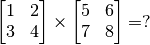）；
• 函数的导数多元函数求导 （测试题：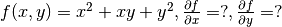）；
• 线性回归
• 梯度下降方法 求函数的局部最小值。

## TensorFlow 1+1¶

import tensorflow as tf
tf.enable_eager_execution()

a = tf.constant(1)
b = tf.constant(1)
c = tf.add(a, b)    # 也可以直接写 c = a + b，两者等价

print(c)

A = tf.constant([[1, 2], [3, 4]])
B = tf.constant([[5, 6], [7, 8]])
C = tf.matmul(A, B)

print(C)


tf.Tensor(2, shape=(), dtype=int32)
tf.Tensor(
[[19 22]
[43 50]], shape=(2, 2), dtype=int32)


import tensorflow as tf
tf.enable_eager_execution()

x = tf.get_variable('x', shape=, initializer=tf.constant_initializer(3.))
y = tf.square(x)


[array([9.], dtype=float32), array([6.], dtype=float32)]


X = tf.constant([[1., 2.], [3., 4.]])
y = tf.constant([[1.], [2.]])
w = tf.get_variable('w', shape=[2, 1], initializer=tf.constant_initializer([[1.], [2.]]))
b = tf.get_variable('b', shape=, initializer=tf.constant_initializer([1.]))
L = 0.5 * tf.reduce_sum(tf.square(tf.matmul(X, w) + b - y))


[62.5, array([[35.],
[50.]], dtype=float32), array([15.], dtype=float32)]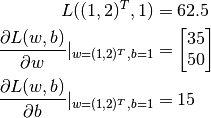## 基础示例：线性回归¶

 年份 2013 2014 2015 2016 2017 房价 12000 14000 15000 16500 17500

import numpy as np

X_raw = np.array([2013, 2014, 2015, 2016, 2017], dtype=np.float32)
y_raw = np.array([12000, 14000, 15000, 16500, 17500], dtype=np.float32)

X = (X_raw - X_raw.min()) / (X_raw.max() - X_raw.min())
y = (y_raw - y_raw.min()) / (y_raw.max() - y_raw.min())


• 初始化自变量为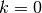• 迭代进行下列步骤直到满足收敛条件：

• 求函数关于自变量的梯度• 更新自变量：。这里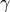是学习率（也就是梯度下降一次迈出的“步子”大小）
•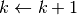### NumPy¶

a, b = 0, 0

num_epoch = 10000
learning_rate = 1e-3
for e in range(num_epoch):
# 手动计算损失函数关于自变量（模型参数）的梯度
y_pred = a * X + b

# 更新参数
a, b = a - learning_rate * grad_a, b - learning_rate * grad_b

print(a, b)


• 经常需要手工求函数关于参数的偏导数。如果是简单的函数或许还好，但一旦函数的形式变得复杂（尤其是深度学习模型），手工求导的过程将变得非常痛苦，甚至不可行。

### TensorFlow¶

TensorFlow的 Eager Execution（动态图）模式  与上述NumPy的运行方式十分类似，然而提供了更快速的运算（GPU支持）、自动求导、优化器等一系列对深度学习非常重要的功能。以下展示了如何使用TensorFlow计算线性回归。可以注意到，程序的结构和前述NumPy的实现非常类似。这里，TensorFlow帮助我们做了两件重要的工作：

• 使用 tape.gradient(ys, xs) 自动计算梯度；
• 使用 optimizer.apply_gradients(grads_and_vars) 自动更新模型参数。
X = tf.constant(X)
y = tf.constant(y)

a = tf.get_variable('a', dtype=tf.float32, shape=[], initializer=tf.zeros_initializer)
b = tf.get_variable('b', dtype=tf.float32, shape=[], initializer=tf.zeros_initializer)
variables = [a, b]

num_epoch = 10000
for e in range(num_epoch):
y_pred = a * X + b
loss = 0.5 * tf.reduce_sum(tf.square(y_pred - y))
# TensorFlow自动计算损失函数关于自变量（模型参数）的梯度
# TensorFlow自动根据梯度更新参数

  Python中可以使用整数后加小数点表示将该整数定义为浮点数类型。例如 3. 代表浮点数 3.0。
  此处的损失函数为均方差。其关于参数 a 和 b 的偏导数为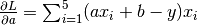，